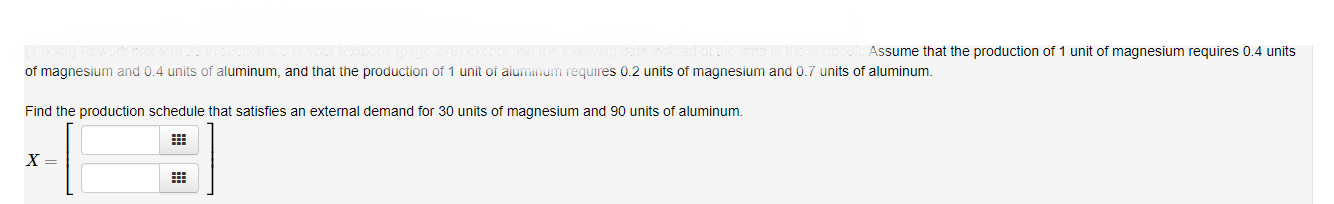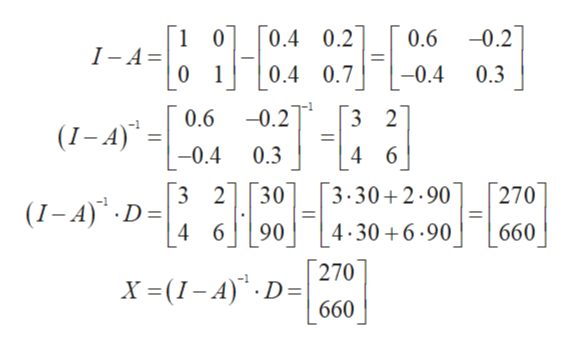# Assume that the production of 1 unit of magnesium requires 0.4 unitsof magnesium and 0.4 units of aluminum, and that the production of 1 unit of aiuminum requires 0.2 units of magnesium and 0.7 units of aluminumFind the production schedule that satisfies an external demand for 30 units of magnesium and 90 units of aluminum.X =

Question
70 views

Assume that the production of 1 unit of magnesium requires 0.4 units of magnesium and 0.4 units of aluminum, and that the production of 1 unit of aluminum requires 0.2 units of magnesium and 0.7 units of aluminum.

Find the production schedule that satisfies an external demand for 30 units of magnesium and 90 units of aluminum.help_outlineImage TranscriptioncloseAssume that the production of 1 unit of magnesium requires 0.4 units of magnesium and 0.4 units of aluminum, and that the production of 1 unit of aiuminum requires 0.2 units of magnesium and 0.7 units of aluminum Find the production schedule that satisfies an external demand for 30 units of magnesium and 90 units of aluminum. X = fullscreen
check_circle

Step 1

First construct a matrix A,

Step 2

By help of the matrix B find the production schedule matrix X, which is given by

X = (I – A)-1 * D

First find the matrix (I – A), then find its inve...help_outlineImage Transcriptionclose-0.2 0.4 0.2 1 0 I - A= 0 1 0.6 0.3 0.4 0.7 -0.4 (-A) 0.6 -0.2 3 2 -0.4 0.3 4 6 270 3.30 2.90 30 3 2 (1-A) D= 4-30 6.90 4 6 90 660 270 X(I-A) D 660 fullscreen

### Want to see the full answer?

See Solution

#### Want to see this answer and more?

Solutions are written by subject experts who are available 24/7. Questions are typically answered within 1 hour.*

See Solution
*Response times may vary by subject and question.
Tagged in

### Math